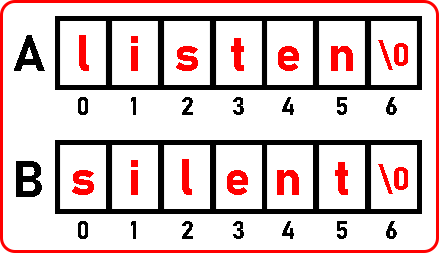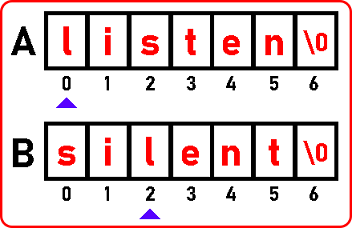# How to Check if 2 Strings are Anagram in C

## How to Check if 2 Strings are Anagram in C Language

In this article, I am going to discuss How to Check if 2 Strings are Anagram in C Language with Examples. Please read our previous article where we discussed Finding Duplicates in a String using Bitwise Operations in C Language with Examples.

##### Checking if 2 Strings are Anagram in C Language:

In this article, we will see how to check if two strings are anagrams or not. First, let’s understand what is meant by anagram? An anagram is the two sets of strings that are formed using the same set of alphabets.For example, here we have a word that is a ‘listen’ and the same alphabets are used in other words which is ‘silent’. So, these are anagrams. Now we have to check whether two strings are anagrams or not. So, the first and foremost thing is to check whether two strings are of equal size. If they are different sizes, they cannot be anagrams. Now how do we check whether the strings are having the same set of letters?

##### 1st Method of Checking if 2 Strings are Anagram or Not

One simple approach takes the alphabet from the 1st string and searches that in the 2nd string.Here we found ‘l’,Now we found ‘i’,Here we found ‘s’,Here we found ‘t’,Now we have found ‘e’,Here we found ‘n’,Now we have to stop scanning the first string as we reached ‘\0’. In this manner, we have compared all the elements and we got the result is the given two strings are anagrams. If any one of the letters of the 1st string is not found in the 2nd string, then we can say that they are not anagrams.

###### So how much time does this procedure take?

We are comparing all the letters of the 1st string with every letter in the 2nd string, so this is O (n2).

Time Complexity: O(n2)

The procedure we have shown you is the simplest procedure and it takes n2 time. This is a time-consuming procedure. One more thing we have to take care that there are no duplicates in both the string. We have not taken any duplicate alphabet if there are any duplicates then we have to deal with that complexity.

So, we have already learned about the counting number of duplicates in an array. That same logic will apply here if there are any duplicates in the given string. Now let us look at the 2nd method and the second method also we are familiar with it which is using a hash table.

##### 2nd Method of Checking if 2 Strings are Anagram or NotWe have taken an array ‘H’ of size 26 because the total number of alphabets is 26 that’s why we are taking this size array. And we already know how to use a hash table as we see in our previous articles.

Here we are taking all the alphabet in lowercases. If we want uppercase and special characters also then we will take an array of size 128. But as an example, we are taking only lowercase means size of the hash table is 26.

Let us use a hash table how we can find whether any two strings are anagrams or not. Let’s have a look at the procedure. First of all, we will write the ASCII codes of these lowercase alphabets.We have written ASCII codes of letters on the top of the array ‘A’. Scan through this string, we will be using a for loop we can go to each and every alphabet. Then for every alphabet, we will subtract 97 from each alphabet’s ASCII code,

For ‘l’, 108 – 97 = 11
For ‘i’, 105 – 97 = 8
For ‘s’, 115 – 97 = 18
For ‘t’, 116 – 97 = 19
For ‘e’, 101 – 97 = 4
For ‘n’, 110 – 97 = 13

Now, the procedure is first subtracted 97 from a particular alphabet’s ASCII code and then increment that index in ‘H’ which we get from subtraction. As we did subtraction above, now increment those indices in ‘H’ one by one. Here we are showing you the final incremented ‘H’ array as we discussed before:This procedure we have already seen earlier for finding duplicates in strings. So, see all these alphabets are unique. There are no duplicates. Suppose if any character is repeating then it will increment and it will become 2.

Now, what is the next step? The next step is to scan through the 2nd string and for each character like we did above, subtract 97 from each alphabet ASCII code, and whatever we get from subtraction increment that index in ‘H’ but here decrements the index value in ‘H’. For example, our 2nd string is ‘silent’,

For ‘s’, 115 – 97 = 18
For ‘i’, 105 – 97 = 8
For ‘l’, 108 – 97 = 11
For ‘e’, 101 – 97 = 4
For ‘n’, 110 – 97 = 13
For ‘t’, 116 – 97 = 19

Now we have to decrement the above indices in array ‘H’. After decrementing in ‘H’, we will get:See any index should not become -1. If it is becoming -1 means that the alphabet is not there. So, we can stop there after subtracting f it becomes -1. If we never got a -1 value it means all the characters are available here. So, these two strings are anagrams. If we got a -1 one, we can stop there.

So, this is the procedure by using one string, we can maintain count in the hash table, and by second string, we can go on documenting it if any number is reducing below 0 that is becoming -1 means it is not found and we can stop there. And otherwise, we can scan for this array once more and check that is all element 0. If anything is not zero you can stop and print these are not anagrams.

Now let us do some analysis of how much time it is taking we are scanning for strings.

For scanning 1st string, it takes n time,

For scanning 2nd string, it will take n time,

We are not accessing the whole hash table; we are accessing a particular location. So, we can neglect this time but here let this time be n.

Time Complexity: O (n + n + n) = O (3n) = O (n)

Now let see the code part.

##### Checking if 2 Strings are Anagram Code in C Language:
#include <stdio.h>
#include <stdlib.h>
int main ()
{
char A[] = “listen”;
char B[] = “silent”;
int i, H;
printf (“Strings \”%s\” and \”%s\””, A, B);
for (i = 0; i < 26; i++)
{
H[i] = 0;
}
for (i = 0; A[i] != ‘\0’; i++)
{
H[A[i] – 97] += 1;
}
for (i = 0; B[i] != ‘\0’; i++)
{
H[B[i] – 97] -= 1;
if (H[B[i] – 97] < 0)
{
printf (” are not anagrams”);
break;
}
}
if (B[i] == ‘\0’)
printf (” are anagrams”);
}

Output: